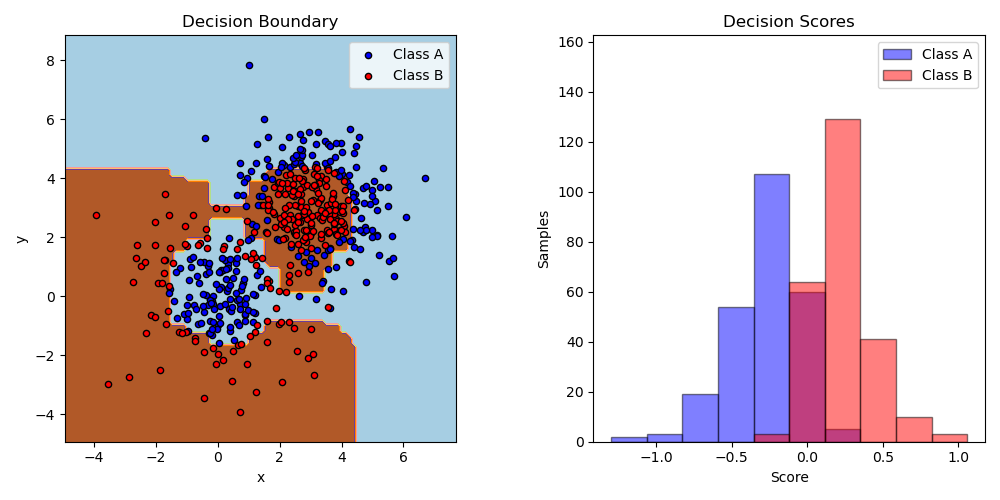This example fits an AdaBoosted decision stump on a non-linearly separable classification dataset composed of two “Gaussian quantiles” clusters (see sklearn.datasets.make_gaussian_quantiles) and plots the decision boundary and decision scores. The distributions of decision scores are shown separately for samples of class A and B. The predicted class label for each sample is determined by the sign of the decision score. Samples with decision scores greater than zero are classified as B, and are otherwise classified as A. The magnitude of a decision score determines the degree of likeness with the predicted class label. Additionally, a new dataset could be constructed containing a desired purity of class B, for example, by only selecting samples with a decision score above some value.# Author: Noel Dawe <noel.dawe@gmail.com>
#

import numpy as np
import matplotlib.pyplot as plt

from sklearn.tree import DecisionTreeClassifier
from sklearn.datasets import make_gaussian_quantiles
from sklearn.inspection import DecisionBoundaryDisplay

# Construct dataset
X1, y1 = make_gaussian_quantiles(
cov=2.0, n_samples=200, n_features=2, n_classes=2, random_state=1
)
X2, y2 = make_gaussian_quantiles(
mean=(3, 3), cov=1.5, n_samples=300, n_features=2, n_classes=2, random_state=1
)
X = np.concatenate((X1, X2))
y = np.concatenate((y1, -y2 + 1))

# Create and fit an AdaBoosted decision tree
DecisionTreeClassifier(max_depth=1), algorithm="SAMME", n_estimators=200
)

bdt.fit(X, y)

plot_colors = "br"
plot_step = 0.02
class_names = "AB"

plt.figure(figsize=(10, 5))

# Plot the decision boundaries
ax = plt.subplot(121)
disp = DecisionBoundaryDisplay.from_estimator(
bdt,
X,
cmap=plt.cm.Paired,
response_method="predict",
ax=ax,
xlabel="x",
ylabel="y",
)
x_min, x_max = disp.xx0.min(), disp.xx0.max()
y_min, y_max = disp.xx1.min(), disp.xx1.max()
plt.axis("tight")

# Plot the training points
for i, n, c in zip(range(2), class_names, plot_colors):
idx = np.where(y == i)
plt.scatter(
X[idx, 0],
X[idx, 1],
c=c,
cmap=plt.cm.Paired,
s=20,
edgecolor="k",
label="Class %s" % n,
)
plt.xlim(x_min, x_max)
plt.ylim(y_min, y_max)
plt.legend(loc="upper right")

plt.title("Decision Boundary")

# Plot the two-class decision scores
twoclass_output = bdt.decision_function(X)
plot_range = (twoclass_output.min(), twoclass_output.max())
plt.subplot(122)
for i, n, c in zip(range(2), class_names, plot_colors):
plt.hist(
twoclass_output[y == i],
bins=10,
range=plot_range,
facecolor=c,
label="Class %s" % n,
alpha=0.5,
edgecolor="k",
)
x1, x2, y1, y2 = plt.axis()
plt.axis((x1, x2, y1, y2 * 1.2))
plt.legend(loc="upper right")
plt.ylabel("Samples")
plt.xlabel("Score")
plt.title("Decision Scores")

plt.tight_layout()